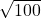Solve the following quadratic function by utilizing the square root method. y = 100 – x2

Question

by utilizing the square root method.
y = 100 – x2

in progress 0
6 months 2021-07-19T16:14:33+00:00 1 Answers 4 views 0

x = ± 10

Step-by-step explanation:

To solve the equation let y = 0 , that is

0 = 100 – x²( add x² to both sides )

x² = 100 (I take the square root of both sides )

x = ±= ± 10

Then solution is x = – 10, x = 10